# Object Detections

Here is the comparison of the most popular object detection frameworks.# R-CNN

Use selective search to generate region proposal, extract patches from those proposal and apply image classification algorithm.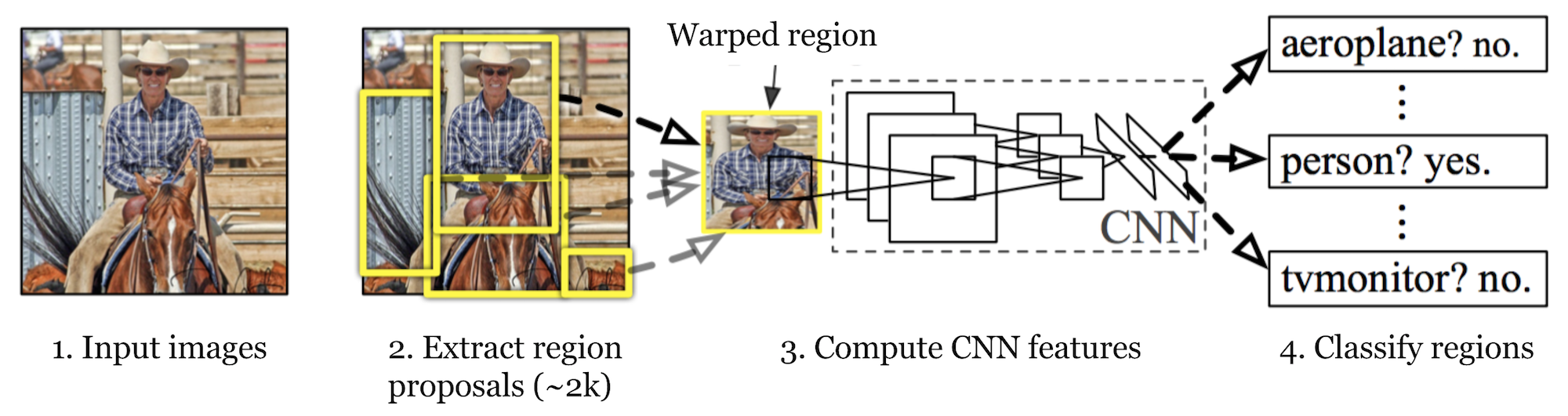# Fast R-CNN

Apply CNN on image then use ROI pooling layer to convert the feature map of ROI to fix length for future classification. Note it still requires an external region proposal generator.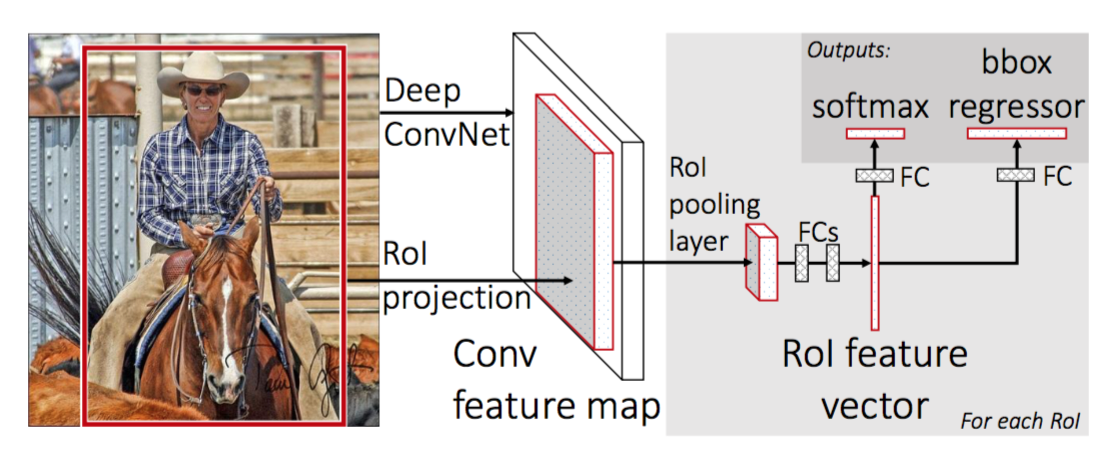# Faster R-CNN

Combine region proposal network and classification CNN, which share the same feature extraction layer. RPN generates region proposals and confidence for each anchor point (e.g., regular grid) and classification CNN apply ROI pooling layer for those proposal as well.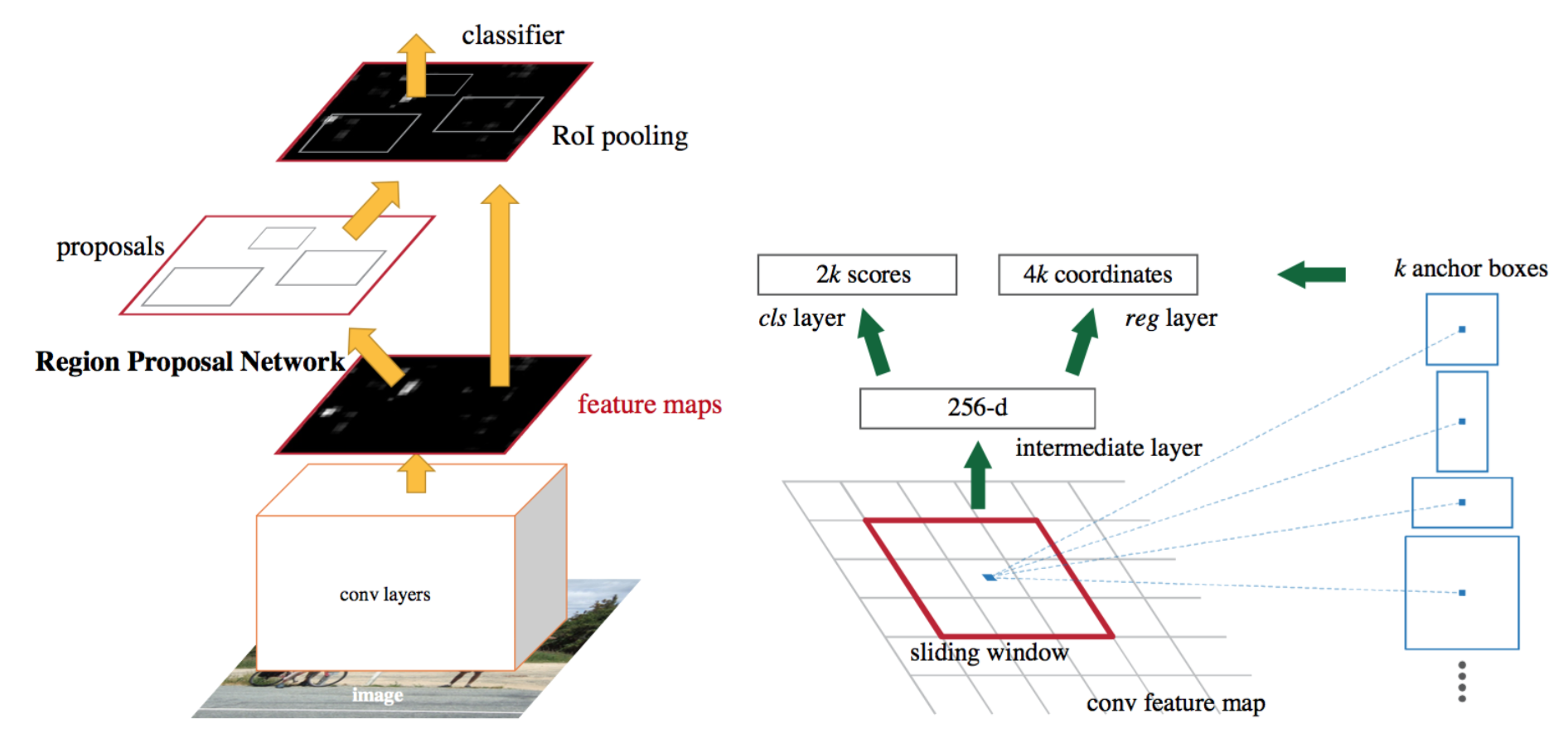Similar to Faster RCNN, but add a branch to generate a binary mask for segmentation for each ROI.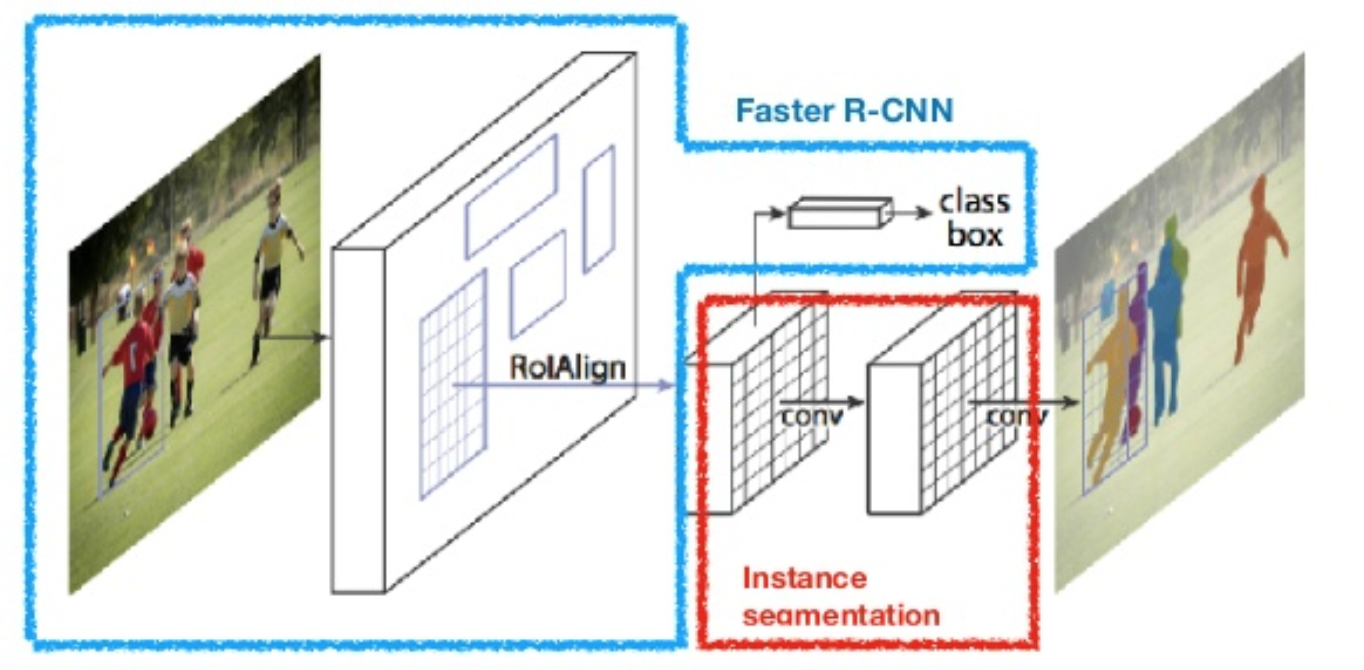# YOLO

Similar to Faster RCNN, the network generates a lot of region proposal (from regular grid) and its confidence, then apply non-maximal suppression.# R-FCN

Similar to Faster RCNN, but it apply ROI pooling layer at the very end of network and the output of that layer is directly the classification probability.# SSD

Like YOLO, but it use fuse the response from not only the last convolution layer but also layers before them.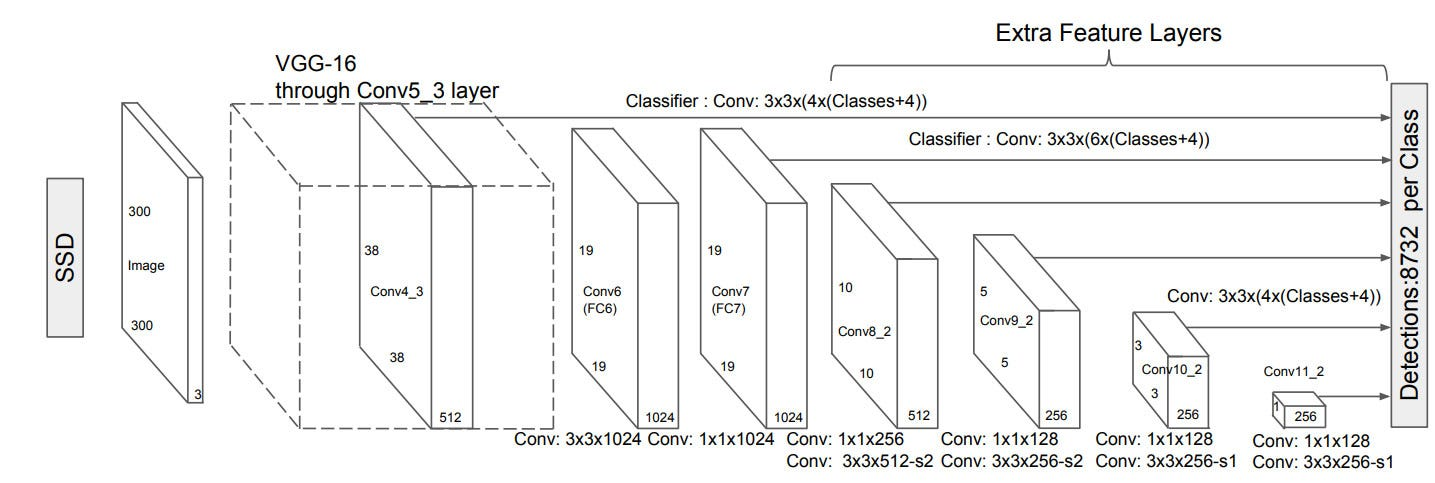# RetinaNet

Focal Loss for Dense Object Detection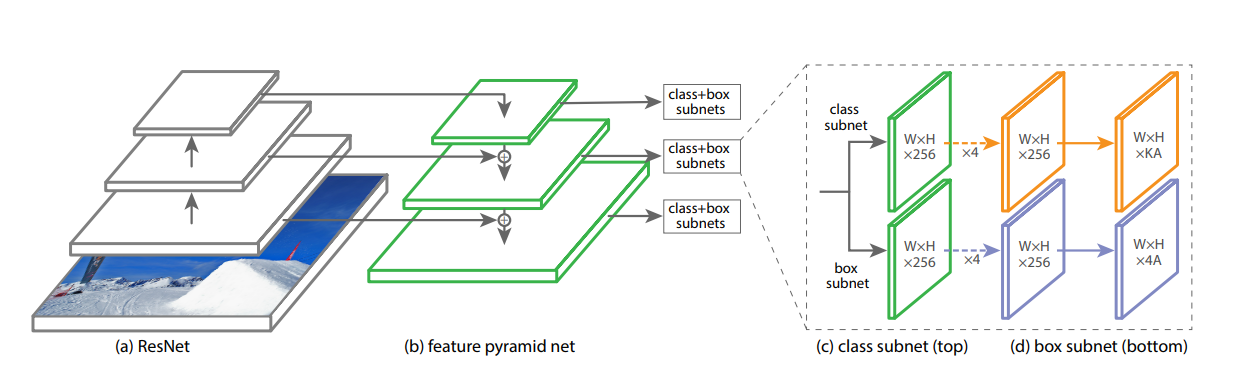The highest accuracy object detectors to date are based on a two-stage approach popularized by R-CNN, where a classifier is applied to a sparse set of candidate object locations. In contrast, one-stage detectors that are applied over a regular, dense sampling of possible object locations have the potential to be faster and simpler, but have trailed the accuracy of two-stage detectors thus far. In this paper, we investigate why this is the case. We discover that the extreme foreground-background class imbalance encountered during training of dense detectors is the central cause.

We propose to address this class imbalance by reshaping the standard cross entropy loss such that it down-weights the loss assigned to well-classified examples. The focal loss function can be written as:

$$FL(p_t)=-\alpha_t(1-p_t)^\gamma log(p_t)$$ where $p_t=p$ for $y=1$ otherwise $p_t=1-p$. For an easy example, $p_t\approx 1$, thus doesn’t affect $FL(p_t)$. $\gamma=2$ and $\alpha=0.5$ is the typical choice.

Feature Pyramid Network is used as the backbone.

# FCOS

Fully Convolutional One-Stage Object DetectionFCOS is anchor-box free, as well as proposal free. FCOS works by predicting a 4D vector (l, t, r, b) encoding the location of a bounding box at each foreground pixel (supervised by ground-truth bounding box information during training).

This done in a per-pixel prediction way, i.e., for each pixel, the network try to predict a bounding box from it, together with the label of class. To counter for the pixel which are far from the ground truth object (center), a centerness score is also predicted which downweights the prediction for those pixels.

If a location falls into multiple bounding boxes, it is considered as an ambiguous sample. For now, we simply choose the bounding box with minimal area as its regression target.

Feature Pyramid Network is used as the backbone.

# Objects as PointsThis paper proposes a CenterNet, which formulates the object detection problem into the problem of detection the center of object and their size of the bounding box is then inferred from the neighbor around the center.

# CornerNet-LiteCornerNet is yet another a single-stage object detection algorithm. CornerNet detects and groups the top-left and bottom-right corners of bounding boxes; it uses a stacked hourglass network to predict the heatmaps of the cor- ners and then uses associate embeddings to group them.

To improve the efficiency of CornerNet, CornerNet-Lite combines two of its variants, CornerNet-Saccade, which uses an attention mechanism to eliminate the need for exhaustively processing all pixels of the image, and CornerNet-Squeeze, which introduces a new compact backbone architecture.

CornerNet-Saccade compute three attention maps for predicting locations of objects at three scales: small (<32), medium (32~96) and lare (>96). The attention maps are generated from multi-scale of a hourglass network.

Written on April 2, 2019